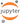# Pseudo Facebook Data - Exploring Two Variables

In this section, we will be re-using the data from the previous post based on Pseudo Facebook data from udacity.

The data from the project corresponds to a typical data set at Facebook. You can load the data through the following command. Notice that this is a TAB delimited csv file. This data set consists of 99000 rows of data. We will see the details of different columns using the command below.

In :
import pandas as pd
import numpy as np
#Read csv file
pf = pd.readcsv("https://s3.amazonaws.com/udacity-hosted-downloads/ud651/pseudofacebook.tsv", sep = '\t')
cats = ['userid', 'dobday', 'dobyear', 'dob_month']
for col in pf.columns:
if col in cats:
pf[col] = pf[col].astype('category')
#summarize data
pf.describe(include='all', percentiles=[]).T.replace(np.nan,' ', regex=True)

/usr/lib/python3.5/site-packages/numpy/lib/function_base.py:3834: RuntimeWarning: Invalid value encountered in percentile
RuntimeWarning)

Out:
count unique top freq mean std min 50% max
userid 99003.0 99003 2.19354e+06 1
age 99003.0 37.2802 22.5897 13 28 113
dob_day 99003.0 31 1 7900
dob_year 99003.0 101 1995 5196
dob_month 99003.0 12 1 11772
gender 98828.0 2 male 58574
tenure 99001.0 537.887 457.65 0 3139
friend_count 99003.0 196.351 387.304 0 82 4923
friendships_initiated 99003.0 107.452 188.787 0 46 4144
likes 99003.0 156.079 572.281 0 11 25111
likes_received 99003.0 142.689 1387.92 0 8 261197
mobile_likes 99003.0 106.116 445.253 0 4 25111
mobile_likes_received 99003.0 84.1205 839.889 0 4 138561
www_likes 99003.0 49.9624 285.56 0 0 14865
www_likes_received 99003.0 58.5688 601.416 0 2 129953

Usually, it is best to use a scatter plot to analyze two variables:

In :
import matplotlib.pyplot as plt
import seaborn as sns
sns.set(style="darkgrid")
%matplotlib inline
ax = sns.regplot(x='age', y='friendcount', data=pf, fitreg=False)
plt.xlim(13, 90)
plt.ylim(0,5000)

Out:
(0, 5000)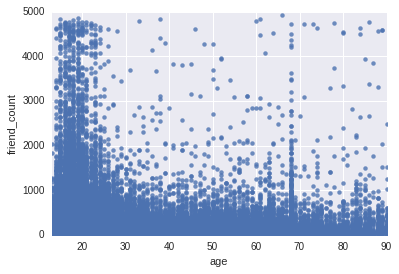We can notice some really interesting behavior in the ugly scatter plot above:

1. The age data is binned, as expected (only integers allowed)
2. Young people around the age of 20 have maximum friend count.
3. There is unusual spike in friends count of people aged more than 100. This could mostly be some flaw in the data, probably based on incorrect entry by the users.
4. People around the age of 70 too have quite a large amount of friends. This is pretty interesting and could point to the use of the social media site by an unexpected group of people.

We can use summary command to find the bounds on age and then use that to limit age axis.

In :
pf.age.describe()

Out:
count    99003.000000
mean        37.280224
std         22.589748
min         13.000000
25%         20.000000
50%         28.000000
75%         50.000000
max        113.000000
Name: age, dtype: float64

Furthermore, we notice at some areas of the plot being too dense, where as some to be really sparse. The areas where points are too dense is called “over plotting” - It is impossible to extract any meaningful statistics from this region. In order to overcome this, we can set the transparency of the plots using the alpha parameter in the plt.scatter() method. Using a value of 1/20 means, one point that will plotted will be equal to 20 original points.

In :
ax = sns.regplot(x='age', y='friend_count', data=pf, fit_reg=False, color='green', scatter_kws={'alpha': 0.05})
plt.xlim(13, 90)
plt.ylim(0,5000)
plt.plot([13, 90], [600, 600], linewidth=2, color='r')

Out:
[<matplotlib.lines.Line2D at 0x7f07d45a22e8>]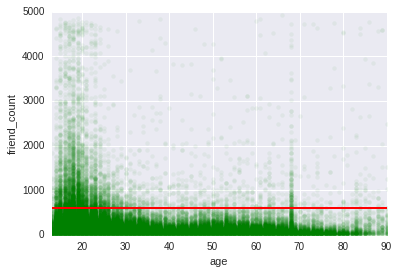Based on these new plots, we can find bulk of higher friend count for younger people is still less than 600. We still find higher count for age group of 70.

Furthermore, we can do a better representation of data using the coord_trans() method. We will be using a square root function.

In order to that, we will first create an "sqrt" scale.

In :
import numpy as np
from numpy import ma
from matplotlib import scale as mscale
from matplotlib import transforms as mtransforms
from matplotlib.ticker import AutoLocator
class SqrtScale(mscale.ScaleBase):
"""
Scales data using np.sqrt method.
The scale function:
np.sqrt(x)
The inverse scale function:
x**2
"""

# The scale class must have a member name that defines the
# string used to select the scale.  For example,
# gca().set_yscale("mercator") would be used to select this
# scale.
name = 'sqrt'
def __init__(self, axis):
"""
Any keyword arguments passed to set_xscale and
set_yscale will be passed along to the scale's
constructor.
"""
mscale.ScaleBase.__init__(self)
def get_transform(self):
"""
Override this method to return a new instance that does the
actual transformation of the data.
The SqrtTransform class is defined below as a
nested class of this one.
"""
return self.SqrtTransform()
def set_default_locators_and_formatters(self, axis):
"""
Override to set up the locators and formatters to use with the
scale.
"""
axis.set_major_locator(AutoLocator())
class SqrtTransform(mtransforms.Transform):
# There are two value members that must be defined.
# input_dims and output_dims specify number of input
# dimensions and output dimensions to the transformation.
# These are used by the transformation framework to do some
# error checking and prevent incompatible transformations from
# being connected together.  When defining transforms for a
# scale, which are, by definition, separable and have only one
# dimension, these members should always be set to 1.
input_dims = 1
output_dims = 1
is_separable = True
def __init__(self):
mtransforms.Transform.__init__(self)
def transform_non_affine(self, a):
"""
This transform takes an Nx1 numpy array and returns a
transformed copy.
"""
return np.sqrt(a)
def inverted(self):
"""
Override this method so matplotlib knows how to get the
inverse transform for this transform.
"""
return SqrtScale.InvertedSqrtTransform()

class InvertedSqrtTransform(mtransforms.Transform):
input_dims = 1
output_dims = 1
is_separable = True

def __init__(self):
mtransforms.Transform.__init__(self)

def transform_non_affine(self, a):
return a**2

def inverted(self):
return SqrtScale.SqrtTransform()
# Now that the Scale class has been defined, it must be registered so
# that matplotlib can find it.
mscale.register_scale(SqrtScale)

In :
fig, ax = plt.subplots()
fig.set_size_inches(8.6, 6.4)
ax = sns.regplot(x='age', y='friend_count', data=pf, fit_reg=False, color='cyan', scatter_kws={'alpha': 0.05}, ax=ax)
plt.xlim(13, 90)
plt.ylim(0,4599)
plt.yscale('sqrt')
plt.plot([13, 90], [600, 600], linewidth=2, color='r')

Out:
[<matplotlib.lines.Line2D at 0x7f07d24f5588>]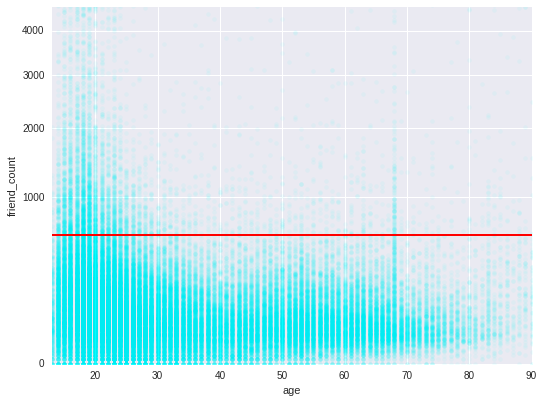On a similar way, we can look at relationship between friends initiated and age.

In :
fig, ax = plt.subplots()
fig.set_size_inches(8.6, 6.4)
kws = {'alpha': 0.05}
ax = sns.regplot(x='age', y='friendships_initiated', data=pf, fit_reg=False, color='purple', scatter_kws=kws, ax=ax)
plt.xlim(13, 90)
plt.ylim(0,4599)
plt.yscale('sqrt')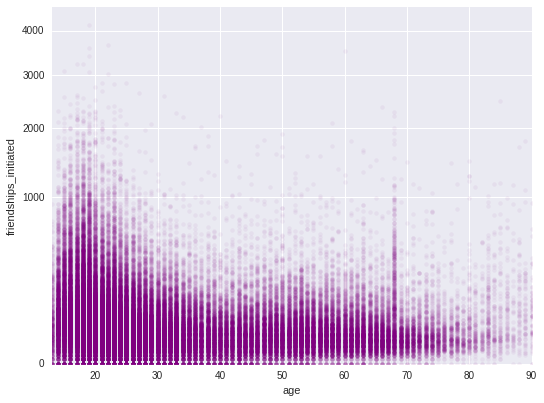Interestingly, we find this distribution to be very similar to the one for friend count.

Scatter plots try to keep us very close to the data. It represents each and every data point. However, in order to judge the quality of a data, it is important to know its important statistics like mean, median etc. How does average of a variable vary wrt to the some other variable.

We want to say, study how does average friend count vary with age. In order to study this we will use the grouping properties of pandas module.

First we want to group our data frame by age. Then, we can create a new data frame that lists friend count mean, median and frequency (n) by using first the groupby() and then using the agg() method. We can look at first few data points of this new data frame using the head() method.

In :
def groupByStats(pf, groupCol, statsCol):
''' return a dataframe with groupByCol'''

# Define the aggregation calculations
aggregations = {

statsCol: {
(statsCol+'_mean'): 'mean',
(statsCol+'_median'): 'median',
(statsCol+'_q25'): lambda x: np.percentile(x,25),
(statsCol+'_q75'): lambda x: np.percentile(x,75),
'n': 'count'/div>
}

}
pf_group_by_age = pf.groupby(groupCol, as_index=False).agg(aggregations).rename(columns = {'':groupCol})
pf_group_by_age.columns = pf_group_by_age.columns.droplevel()
return pf_group_by_age

pf_group_by_age = groupByStats(pf, 'age', 'friend_count')
pf_group_by_age.head(20)

Out:
age friend_count_q75 n friend_count_mean friend_count_median friend_count_q25
0 13 230.00 484 164.750000 74.0 23.75
1 14 293.00 1925 251.390130 132.0 44.00
2 15 377.50 2618 347.692131 161.0 55.00
3 16 385.75 3086 351.937135 171.5 63.00
4 17 360.00 3283 350.300640 156.0 56.00
5 18 368.00 5196 331.166282 162.0 55.00
6 19 350.00 4391 333.692097 157.0 59.00
7 20 304.00 3769 283.499071 135.0 49.00
8 21 265.00 3671 235.941160 121.0 42.00
9 22 239.00 3032 211.394789 106.0 40.00
10 23 216.00 4404 202.842643 93.0 32.00
11 24 209.50 2827 185.712062 92.0 33.00
12 25 156.00 3641 131.021148 62.0 19.00
13 26 169.00 2815 144.008171 75.0 28.00
14 27 159.00 2240 134.147321 72.0 28.00
15 28 150.00 2364 125.835448 66.0 23.00
16 29 142.25 1936 120.818182 66.0 25.00
17 30 146.00 1716 115.208042 67.5 24.00
18 31 143.00 1694 118.459858 63.0 25.00
19 32 140.00 1443 114.279972 63.0 21.00

Now, let us look at this new data frame visually. We can first look at the relationship between average friend count and age.

In :
pf_group_by_age.plot(x='age', y='friend_count_mean', legend=False)
plt.ylabel("Friend Count Mean")

Out:
<matplotlib.text.Text at 0x7f07d16f9dd8>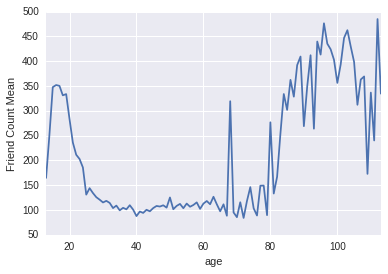We can use this plot as good summary of the original scatter plot and put the two on top of each other.

In :
ax = sns.regplot(x='age', y='friend_count', data=pf, fit_reg=False, color='cyan', x_jitter=0.5, y_jitter=1.0, scatter_kws={'alpha': 0.05})
pf_group_by_age.plot(x='age', y='friend_count_q25', ax=ax, color='red', style='--')
pf_group_by_age.plot(x='age', y='friend_count_median', ax=ax, color='blue')
pf_group_by_age.plot(x='age', y='friend_count_mean', ax=ax, color='green', style='--')
pf_group_by_age.plot(x='age', y='friend_count_q75', ax=ax, color='red')
plt.xlim(13, 70)
plt.ylim(0,1000)
plt.ylabel("Friend Count")

Out:
<matplotlib.text.Text at 0x7f07d091cbe0>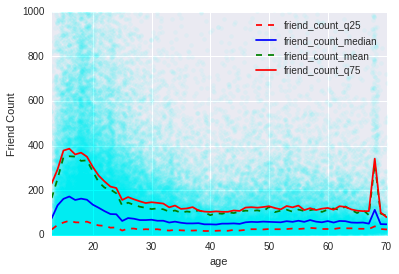In the above plot, we can see that between the age group 30-69, 75% of population has less than 200 friends.

In stead of using 4 different summary measures to analyze the above data, we can use a single number!

Often analysts will use correlation coefficients to summarize this. We will use the Pearson product moment correlation (r). You can look at the pandas corr() method for details. This measures a linear correlation between two variables.

In :
df = pf[(pf['age'] < 70) & (pf['age'] >= 13)]
df['age'].corr(df['friend_count'], method='pearson')

Out:
-0.17121437273281295

We can also have other measures of relationship. For example, a measure of monotonic relationship would be done using Spearman coefficient. Similarly, a measure of strength of dependence between two variables can be done using the “Kendall Rank Coefficient”. A more detailed description about these can be found at https://www.statisticssolutions.com/correlation-pearson-kendall-spearman/.

We will now look at variables that are strictly correlated using scatter plots.

One such example in our dataset would be a relationship between likes_received (y) vs. www_likes_received (x).

In :
ax = sns.regplot(x='www_likes_received', y='likes_received', data=pf, color='cyan', ci=None, line_kws={'color': 'red'})
plt.xlim(0, np.percentile(pf.www_likes_received, 95))
plt.ylim(0, np.percentile(pf.likes_received, 95))

Out:
(0, 561.0)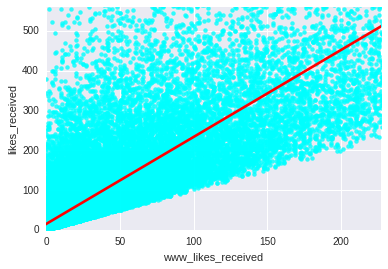We have used numpy percentile() method to find upper limits of x and y data. Additionally, we added a correlation line using the regplot().

We can find the numerical value of the correlation between these two variables.

In :
pf['www_likes_received'].corr(pf['likes_received'], method='pearson')

Out:
0.9479901803455516

Correlation between two variables might not be a good thing always. For example in the above case, it was simply due to the artifact of the two data sets were highly coupled, one was a super set of the other.

Let us take a look again at the modified data frame created using the groupby methods. In particular, we want to remove any noise in the average values.

In :
pf['age_with_months'] = pf.age + (12-pf.dob_month)/12
pf_group_by_age_with_months = groupByStats(pf, 'age_with_months', 'friend_count')
pf1 = pf_group_by_age[pf_group_by_age['age'] < 71]
pf2 = pf_group_by_age_with_months[pf_group_by_age_with_months['age_with_months'] < 71]

In :
f, (ax1, ax2, ax3) = plt.subplots(3)
f.set_size_inches(9, 9)
sns.regplot(x='age_with_months', y='friend_count_mean', data=pf2, scatter=False, lowess=True, ci=95, line_kws={'color': 'red'}, ax=ax1)
pf2.plot(x='age_with_months', y='friend_count_mean', legend=False, ax=ax1)
ax1.set_xlim([13, 71])
sns.regplot(x='age', y='friendcountmean', data=pf1, scatter=False, lowess=True, ci=95, linekws={'color': 'green'}, ax=ax2)
pf1.plot(x='age', y='friendcountmean', legend=False, ax=ax2)
ax2.setxlim([13, 71])
pf11 = pf1.copy()
pf11['ageRounded'] = np.round(pf1['age']/5.0)*5.0
sns.regplot(x='ageRounded', y='friendcountmean', data=pf11, scatter=False, lowess=True, ci=95, linekws={'color': 'cyan'}, ax=ax3)
pf11.plot(x='ageRounded', y='friendcountmean', legend=False, ax=ax3)
ax3.setxlim([13, 71])

Out:
(13, 71)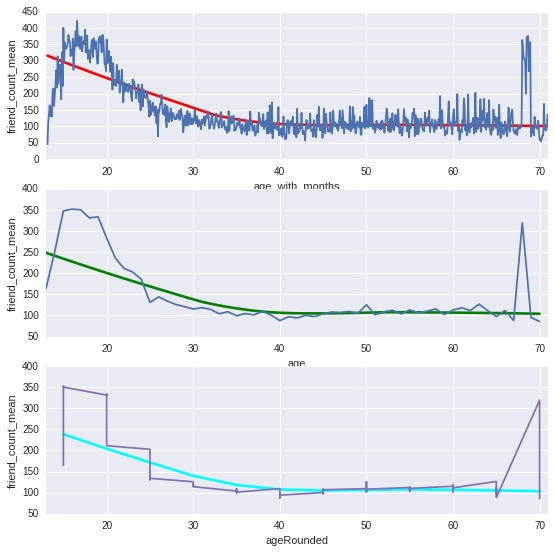This is an example of bias variance trade off, and is similar to the trade off we make when choosing the bin width in histograms. One way, we can do this quite easily in seaborn is using the lowess option, however, in the current implementation it fails to provide any error estimate on the fitted regression!

Lowess option in the seaborn library uses Loess and Lowess method for smoothing. Here, the model is based on the idea that data is continuous and smooth.

So, through this post, we have noticed several ways to plot the same data. The obvious that arises is which plot to choose? In EDA, however, answer to this is, simply you should choose. The idea in EDA is that the same data when plotted differently, glean different incites.

Share This:

## Comments

### Stay in touch

Like the posts you see here? Sign up to get notified about new ones.

# Get The Best Of Posts Delivered To Your Inbox

Subscribe to my newsletter and stay updated.